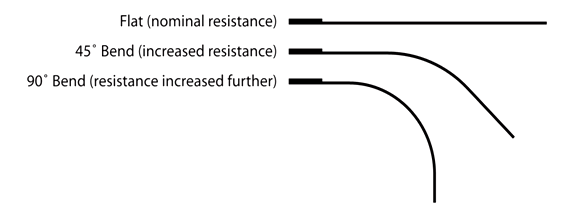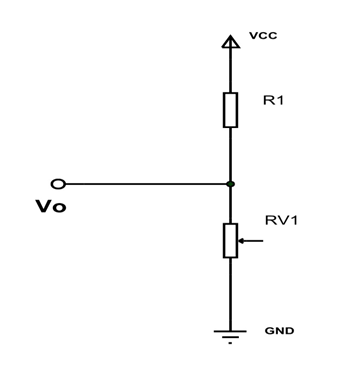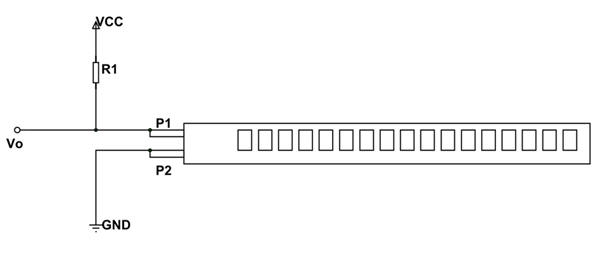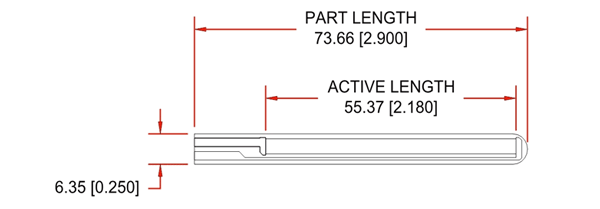# Flex Sensor

Flex sensors are usually available in two sizes. One is 2.2 inch and another is 4.5 inch. Although the sizes are different the basic function remains the same. They are also divided based on resistance. There are LOW resistance, MEDIUM resistance and HIGH resistance types. Choose the appropriate type depending on requirement.  Here we are going to discuss 2.2inch Flex sensor that is FS-L-0055.

### FLEX SENSOR function

FLEX SENSOR terminal resistance changes when it is bent.

### FLEX SENSOR Pin Configuration

Flex sensor is a two terminal device. The Flex sensor does not have polarized terminals like diode. So there is no positive and negative.

 Pin Number Description P1 Usually connected to positive of power source. P2 Usually connected to ground.

### FLEX SENSOR Features and Specifications

• Operating  voltage of FLEX SENSOR: 0-5V
• Can operate on LOW voltages
• Power rating : 0.5Watt (continuous), 1 Watt (peak)
• Life: 1 million
• Operating temperature: -45ºC to +80ºC
• Flat Resistance: 25K Ω
• Resistance Tolerance: ±30%
• Bend Resistance Range: 45K to 125K Ohms(depending on bend)

Note: Complete technical information can be found in the Flex Sensor Datasheet linked at the bottom of this page.

### FS-L-0055 Equivalents

FS-L-0095, FS-L-0112, etc.

### Where to Use FLEX SENSOR

For understanding the use of FLEX SENSOR consider:

Case1: Where you want to check whether the surface of a device or thing is leveled or not. Say you want a device to check whether a window or door is open or not. At that time a Flex sensor could be used. The sensor could be fixed at door edge and when the door opens the Flex sensor gets flexed. With the sensor being flexed its parameters changes which could be designed to provide an alert.

Case2: Where you want to measure the FLEX or BENT or ANGLE change of any instrument or device. The FLEX SENSOR internal resistance changes almost linearly with its flex angle. So by sticking the sensor to the instrument, we can have the flex angle in electrical parameter of resistance.

### How to Use FLEX SENSOR

As mentioned earlier, FLEX SENSOR is basically a VARIABLE RESISTOR whose terminal resistance increases when the sensor is bent. So this sensor resistance increases depends on surface linearity. So it is usually used to sense the changes in linearity.As shown in the above figure, when the surface of FLEX SENSOR is completely linear it will be having its nominal resistance. When it is bent 45º angle the FLEX SENSOR resistance increases to twice as before. And when the bent is 90º the resistance could go as high as four times the nominal resistance. So the resistance across the terminals rises linearly with bent angle. So in a sense the FLEX sensor converts flex angle to RESISTANCE parameter.

For convenience we convert this RESISTANCE parameter to VOLTAGE parameter. For that we are going to use VOLTAGE DIVIDER circuit. A typical VOLTAGE DIVIDER circuit is shown below.In this resistive network we have two resistances. One is constant resistance (R1) and other is variable resistance (RV1). Vo is the voltage at midpoint of VOLTAGE DIVIDER circuit and is also the output voltage. Vo is also the voltage across the variable resistance (RV1). So when the resistance value of RV1 is changed the output voltage Vo also changes. So we will have resistance change in voltage change with VOLTAGE DIVIDER circuit.

Here we will replace the variable resistance (RV1) with FLEX SENSOR. The circuit will be as below.As shown in figure, R1 here is a constant resistance and FLEX SENSOR which acts as a variable resistance. Vo being output voltage and also the voltage across the FLEX SENSOR.

Here,
Vo = VCC (Rx/ (R1+Rx)).
Rx - FLEX SENSOR resistance

Now, when the FLEX SENSOR is bent the terminal resistance increases. This increase also appears in VOLTAGE DIVIDER circuit. With that the drop across the FLEX SENSOR increases so is Vo.  So with increase in bent of FLEX sensor Vo voltage increases linearly. With that we have VOLTAGE parameter representing the flex.

We can take this VOLTAGE parameter and feed it to ADC to get the digital value which can be used conveniently.

### Applications

• Robotics
• Gaming (Virtual Motion)
• Medical Devices
• Computer Peripherals
• Musical Instruments
• Physical Therapy

### 2D-Model and Dimensions

Measurements in millimeter (inches)Component Datasheet

## Related Post

### Join 20K+subscribers

We will never spam you.

* indicates required

Be a part of our ever growing community.